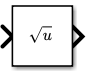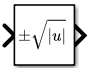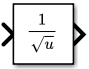# Sqrt

Calculate square root, signed square root, or reciprocal of square root

•Libraries:
HDL Coder / HDL Floating Point Operations
HDL Coder / Math Operations

## Description

The Sqrt block calculates the square root, signed square root, or reciprocal of square root on the input signal. Select one of the following functions from the Function parameter list.

FunctionDescriptionMathematical Expression MATLAB® Equivalent
`sqrt`

Square root of the input

`u0.5`

`sqrt`
`signedSqrt`

Square root of the absolute value of the input, multiplied by the sign of the input

`sign(u)*|u|0.5`

`rSqrt`

Reciprocal of the square root of the input

`u-0.5`

The block icon changes to match the function.

## Ports

### Input

expand all

Input signal to the block to calculate the square root, signed square root, or reciprocal of square root. The `sqrt` function accepts real or complex inputs, except for complex fixed-point signals. `signedSqrt` and `rSqrt` do not accept complex inputs. The input signal must be a floating point number.

This table summarizes the support for complex types and negative values for floating point, integer, and fixed-point data types for `sqrt`, `rSqrt`, and `signedSqrt` functions.

FunctionData TypeComplexNegative Values
InputOutput
`sqrt`Floating pointYesYesYes
Integer and fixed-pointNoNoNo

`rSqrt`

Floating pointNoNoYes
Integer and fixed-pointNoNoNo
`signedSqrt`Floating pointNoYesYes
Integer and fixed-pointNoNoNo

If the input is negative, set the Output signal to complex for all functions except `signedSqrt`.

Data Types: `single` | `double` | `half` | `int8` | `int16` | `int32` | `int64` | `uint8` | `uint16` | `uint32` | `uint64` | `fixed point`

### Output

expand all

Output signal that is the square root, signed square root, or reciprocal of square root of the input signal. When the input is an integer or fixed-point type, the output must be floating point.

Data Types: `single` | `double` | `half` | `int8` | `int16` | `int32` | `int64` | `uint8` | `uint16` | `uint32` | `uint64` | `fixed point`

## Parameters

expand all

### Main

Specify the mathematical function that the block calculates. The block icon changes to match the function you select.

FunctionBlock Icon
`sqrt``signedSqrt``rSqrt`#### Dependency

When this parameter is set to `signedSqrt`, the Intermediate results data type parameter is disabled.

#### Programmatic Use

 Block Parameter: `Operator` Type: character vector Values: `'sqrt'` | `'signedSqrt'` | `'rSqrt'` Default: `'sqrt'`

Specify the output signal type of the block.

FunctionInput Signal TypeOutput Signal Type
AutoRealComplex

`sqrt`

`real`

`real` for nonnegative inputs

`NaN` for negative inputs

`real` for nonnegative inputs

`NaN` for negative inputs

`complex`

`complex`

`complex`

`error`

`complex`

`signedSqrt`

`real`

`real`

`real`

`complex`

`complex`

`error`

`error`

`error`

`rSqrt`

`real`

`real`

`real`

`error`

`complex`

`error`

`error`

`error`

#### Programmatic Use

 Block Parameter: `OutputSignalType` Type: character vector Values: `'auto'` | `'real'` | `'complex'` Default: `'auto'`

Specify the time interval between samples. To inherit the sample time, set this parameter to `-1`. For more information, see Specify Sample Time.

#### Dependencies

This parameter is visible only if you set it to a value other than `-1`. To learn more, see Blocks for Which Sample Time Is Not Recommended.

#### Programmatic Use

 Block Parameter: `SampleTime` Type: string scalar or character vector Default: `"-1"`

### Algorithm

Specify the method for computing the reciprocal of a square root. This parameter is only valid for the `rSqrt` function.

MethodData Types SupportedWhen to Use This Method
`Exact`

Floating point

You do not want an approximation.

Note

The input or output must be floating point.

`Newton-Raphson`

Floating-point, fixed-point, and built-in integer types

You want a fast, approximate calculation.

The `Exact` method provides results that are consistent with MATLAB computations.

Note

The algorithms for `sqrt` and `signedSqrt` are always of `Exact` type, no matter what selection appears on the block dialog box.

#### Programmatic Use

 Block Parameter: `AlgorithmType` Type: character vector Values: `'Exact'` | `'Newton-Raphson'` Default: `'Exact'`

Specify the number of iterations to perform the Newton-Raphson algorithm. This parameter is valid with the `rSqrt` function and the `Newton-Raphson` value for Method.

Note

If you enter 0, the block output is the initial guess of the Newton-Raphson algorithm.

#### Programmatic Use

 Block Parameter: `Iterations` Type: character vector Values: integer Default: `'3'`

### Data Types

The Data Type Assistant helps you set data attributes. To use the Data Type Assistant, click. For more information, see Specify Data Types Using Data Type Assistant.

Specify the data type for intermediate results when you set Function to `sqrt` or `rSqrt` on the Main pane.

The type can be inherited, specified directly, or expressed as a data type object such as `Simulink.NumericType`.

Note

To avoid overflow, the intermediate data type must be larger than or equal to a data type that can contain the square of the output data type.

Follow these guidelines on setting an intermediate data type explicitly for the square root function, `sqrt`:

Input and Output Data TypesIntermediate Data Type
Input or output is double.Use double.
Input or output is single, and any non-single data type is not double. Use single or double.
Input and output are fixed point.Use fixed point.

Follow these guidelines on setting an intermediate data type explicitly for the reciprocal square root function, `rSqrt`:

Input and Output Data TypesIntermediate Data Type
Input is double and output is not single.Use double.
Input is not single and output is double.Use double.
Input and output are fixed point.Use fixed point.

Caution

Do not set Intermediate results data type to `Inherit:Inherit from output` when:

• You select `Newton-Raphson` to compute the reciprocal of a square root.

• The input data type is floating point.

• The output data type is fixed point.

Under these conditions, selecting ```Inherit:Inherit from output``` yields suboptimal performance and produces an error.

To avoid this error, convert the input signal from a floating-point to fixed-point data type. For example, insert a Data Type Conversion block in front of the Sqrt block to perform the conversion.

#### Dependencies

This parameter is disabled when the Function parameter is set to `signedSqrt`.

#### Programmatic Use

 Block Parameter: `IntermediateResultsDataTypeStr` Type: character vector Values: ```'Inherit: Inherit via internal rule'``` | ```'Inherit: Inherit from input'``` | ```'Inherit: Inherit from output'``` | `'double'` | `'single'`, `'int8'`, `'uint8'`, `int16`, `'uint16'`, `'int32'`, `'uint32'`, `'int64'`, `'uint64'`, `fixdt(1,16,0)`, `fixdt(1,16,2^0,0)`. ```''``` Default: ```'Inherit: Inherit via internal rule'```

Specify the output data type. The type can be inherited, specified directly, or expressed as a data type object such as `Simulink.NumericType`.

#### Dependencies

When input is a floating-point data type smaller than single precision, the ```Inherit: Inherit via internal rule``` output data type depends on the setting of the Inherit floating-point output type smaller than single precision configuration parameter. Data types are smaller than single precision when the number of bits needed to encode the data type is less than the 32 bits needed to encode the single-precision data type. For example, `half` and `int16` are smaller than single precision.

#### Programmatic Use

 Block Parameter: `OutDataTypeStr` Type: character vector Values: ```'Inherit: Inherit via internal rule'``` | ```'Inherit: Inherit via back propagation'``` | ```'Inherit: Same as first input'``` | `'double'` | `'single'` | `'half'` | `'int8'` | `'uint8'` | `int16` | `'uint16'` | `'int32'` | `'uint32'` | `'int64'` | `'uint64'` | `fixdt(1,16,0)` | `fixdt(1,16,2^0,0)` | `fixdt(1,16,2^0,0)` | ```''``` Default: ```'Inherit: Same as first input'```

Specify the lower value of the output range that Simulink® checks as a finite, real, double, scalar value.

Note

If you specify a bus object as the data type for this block, do not set the minimum value for bus data on the block. Simulink ignores this setting. Instead, set the minimum values for bus elements of the bus object specified as the data type. For information on the Minimum parameter for a bus element, see `Simulink.BusElement`.

Simulink uses the minimum to perform:

Note

Output minimum does not saturate or clip the actual output signal. Use the Saturation block instead.

#### Programmatic Use

 Block Parameter: `OutMin` Type: character vector Values: scalar Default: ```'[ ]'```

Specify the upper value of the output range that Simulink checks as a finite, real, double, scalar value.

Note

If you specify a bus object as the data type for this block, do not set the maximum value for bus data on the block. Simulink ignores this setting. Instead, set the maximum values for bus elements of the bus object specified as the data type. For information on the Maximum parameter for a bus element, see `Simulink.BusElement`.

Simulink uses the maximum value to perform:

Note

Output maximum does not saturate or clip the actual output signal. Use the Saturation block instead.

#### Programmatic Use

 Block Parameter: `OutMax` Type: character vector Values: scalar Default: ```'[ ]'```

Specify the rounding mode for fixed-point operations. For more information, see Rounding (Fixed-Point Designer).

#### Programmatic Use

 Block Parameter: `RndMeth` Type: character vector Values: ```'Ceiling' | 'Convergent' | 'Floor' | 'Nearest' | 'Round' | 'Simplest' | 'Zero'``` Default: `'Floor'`

Select to lock the output data type setting of this block against changes by the Fixed-Point Tool and the Fixed-Point Advisor. For more information, see Use Lock Output Data Type Setting (Fixed-Point Designer).

#### Programmatic Use

 Block Parameter: `LockScale` Type: character vector Values: `'off'` | `'on'` Default: `'off'`

ActionReasons for Taking This ActionWhat Happens for OverflowsExample

Select this check box.

Your model has possible overflow, and you want explicit saturation protection in the generated code.

Overflows saturate to either the minimum or maximum value that the data type can represent.

The maximum value that the `int8` (signed, 8-bit integer) data type can represent is 127. Any block operation result greater than this maximum value causes overflow of the 8-bit integer. With the check box selected, the block output saturates at 127. Similarly, the block output saturates at a minimum output value of -128.

Do not select this check box.

You want to optimize efficiency of your generated code.

You want to avoid overspecifying how a block handles out-of-range signals. For more information, see Troubleshoot Signal Range Errors.

Overflows wrap to the appropriate value that is representable by the data type.

The maximum value that the `int8` (signed, 8-bit integer) data type can represent is 127. Any block operation result greater than this maximum value causes overflow of the 8-bit integer. With the check box cleared, the software interprets the overflow-causing value as `int8`, which can produce an unintended result. For example, a block result of 130 (binary 1000 0010) expressed as `int8`, is -126.

When you select this check box, saturation applies to every internal operation on the block, not just the output or result. Usually, the code generation process can detect when overflow is not possible. In this case, the code generator does not produce saturation code.

#### Programmatic Use

 Block Parameter: `DoSatur` Type: character vector Value: `'off'` | `'on'` Default: `'off'`

## Block Characteristics

 Data Types `double` | `fixed point` | `half` | `integer` | `single` Direct Feedthrough `yes` Multidimensional Signals `yes` Variable-Size Signals `yes` Zero-Crossing Detection `no`

## Version History

Introduced in R2010a